﻿ SQL Like Statement - TutorialsTrend
This Angular tutorial helps you get started with Angular quickly and effectively through many practical examples.
`

# SQL-Like

To search for a character string using one or more wildcard characters in a LIKE query, simply include the wildcards in a string literal along with the portion of the string. So let's take a look at a practical example of how to use a like operator to search in SQL Server. The example is developed in SQL Server 2019 using the SQL Server Management Studio

#### SQL Server LIKE Clause

The like operator is a logical operator and used in a where clause clause of the SELECT, UPDATE and DELETE statements to search for a specified pattern of characters using the wildcard(Percent sign (%), Underscore (_), Bracket ([]), Caret (^) ) mechanism in a column. Wildcard characters make the like operator more flexible than using = and != (Not Equal To) string comparison operators.

#### Wildcard Characters

There are four types of wildcard characters in SQL Server:

1. Percent sign (%)
2. Underscore (_)
3. Bracket ([])
4. Caret (^)

#### Creating a Table in SQL Server

The table looks as in the following figure: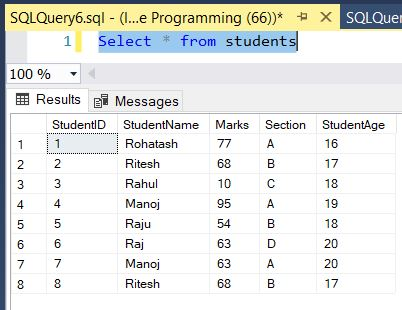Now we perform searching on the above table using wildcard characters.

#### Like with Percent sign (%)

It is used to search any string of zero or more characters.

``````SELECT * FROM students WHERE StudentName LIKE 'ra%' -- return all columns from students table where StudentName name start with 'ra'
SELECT * FROM students WHERE StudentName LIKE '%U' -- return all columns from students table where StudentName name End with 'n'
SELECT * FROM students WHERE StudentName LIKE '%an%'-- return all columns from students table where StudentName name have the letters End with 'an' ``````

The result table will show the following information: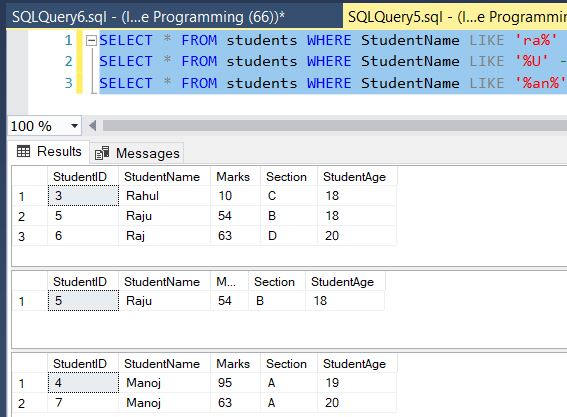#### Like with Underscore (_)

It is used to search for a single character. Here are some examples showing different LIKE operators with "%" and "_" wildcards:

WHERE students LIKE '_p%' Finds any values that have "p" in the second position
WHERE students LIKE 'b_%' Finds any values that start with "b" and are at least 2 characters in length
WHERE students LIKE 'a__%' Finds any values that start with "a" and are at least 3 characters in length

For Example

``````SELECT * FROM students WHERE StudentName LIKE '_a%'
--Finds any values that have "a" in the second position
SELECT * FROM students WHERE StudentName LIKE 'R_%'
--Finds any values that start with "R" and are at least 2 characters in length
SELECT * FROM students WHERE StudentName LIKE 'M__%'
--Finds any values that start with "M" and are at least 3 characters in lengthThe result table will show the following information: ``````

The result table will show the following information: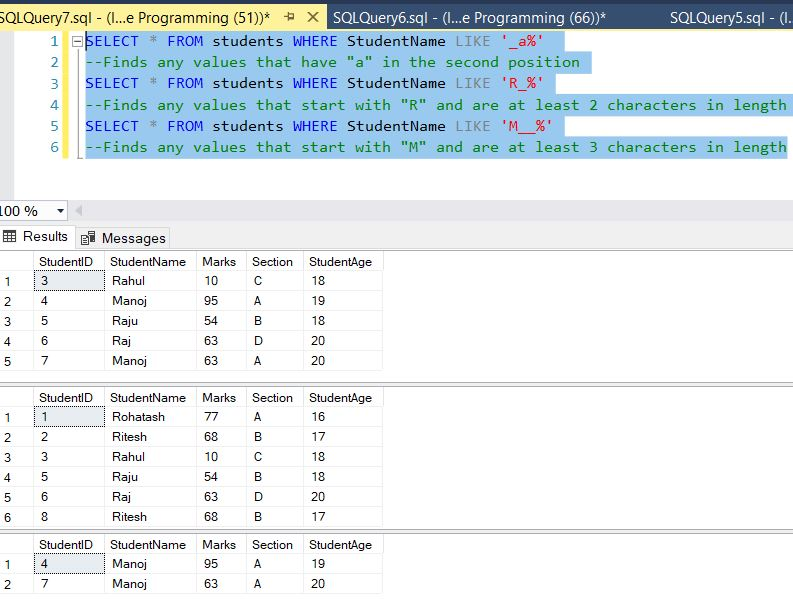#### Like with Bracket ([])

It is used to search for any single character within the specified range.

``````SELECT * FROM students WHERE StudentName like '[rc]%'
--return all columns from students table where StudentName name begin with 'R' or 'C'
SELECT * FROM students WHERE StudentName LIKE '[rc]%u'
--return all columns from students table where StudentName name begin with 'R' or 'C' and end with 'U'.
SELECT * FROM students WHERE StudentName LIKE 'R[a]%'
--return all columns from students table where StudentName name begin with 'R' and hold an 'a' in the second place. ``````

The result table will show the following information: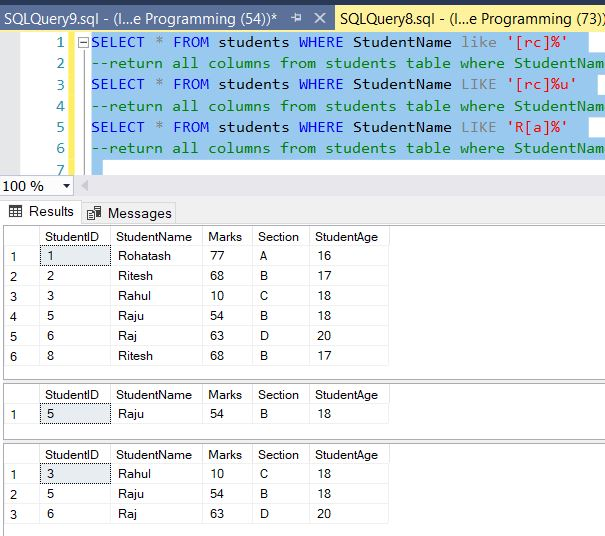#### Like with Caret (^)

It is used to search for any single character that is not the specified character.

``````SELECT * FROM students WHERE StudentName like 'R[^H]%'
--return all columns from UserDetail table where FirstName name begin with 'R' and does not contain an 'H' as the second place
SELECT * FROM students WHERE StudentName like '%A[^P]'
-- return all columns from students table where StudentName name Contain with 'A' and does not contain an 'P' ``````

The result table will show the following information: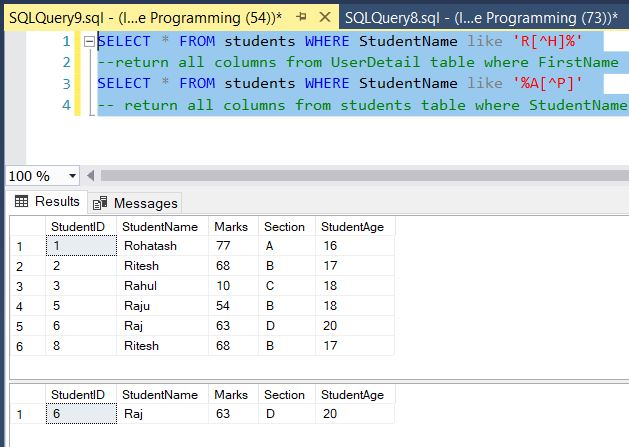#### SQL Server LIKE with ESCAPE

The ESCAPE clause specified that the character ! is the escape character. It instructs the LIKE operator to treat the % character as a literal string instead of a wildcard.

First, create a new table for the demonstration:

``````CREATE TABLE Article(
Article_id INT IDENTITY(1, 1) PRIMARY KEY,
comment VARCHAR(255) NOT NULL
);``````

Second, insert some rows into the Article table:

``````INSERT INTO Article(comment) VALUES('40% discount'),
('40USD off?'),('20% discount today'),('40rs discount today');``````

Third, query data from the Article table:

``SELECT * FROM Article``

Table looks like that: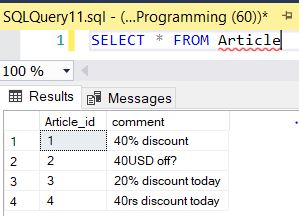If you want to search for 40% in the comment column, you may come up with a query like this:

``````SELECT
Article_id,
comment
FROM
Article
WHERE
comment LIKE '%40%';``````

Table Search result looks like that: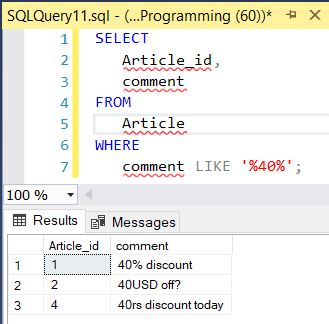The query returns the comments that contain 40% ,40USD and 40rs which is not what we expected.

To solve this issue, you need to use the ESCAPE clause:

Image8

Next